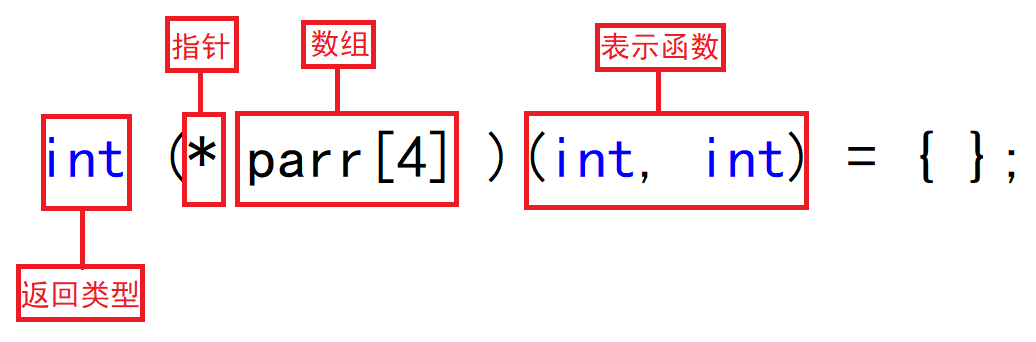#### 数组参数和指针参数

##### 一维数组传参

``````void test(int arr[])
{}
void test(int* arr)
{}
void test1(int* arr1[])
{}
void test1(int** arr1)
{}

int main()
{
int arr[] = { 0 };
int* arr1 = { 0 };
test(arr);
test1(arr1);
return 0;
}    ``````

##### 二维数组传参

1. 二维数组传参时参数可以和主函数中的数组形式保持完全一致。

``````void test(int arr)
{}

int main()
{
int arr = { 0 };
test(arr);
return 0;
}``````

``````void test(int(*arr))
{}

int main()
{
int arr = { 0 };
test(arr);
return 0;
}``````
##### 指针传参

``````void test(int* a)
{}

int main()
{
int a = 0;
int* p = &a;
test(&a);
test(p);
return 0;
}``````

``````void test(int** ptr)
{}
void test1(int** ptr)
{}
void test2(int** ptr)
{}

int main()
{
int a = 0;
int* p = &a;
int** pp = &p;
test(pp);
test1(&p);
int* arr[] = { 0 };
test2(arr);  //数组名是首元素地址，数组首元素是int*类型
return 0;
}``````
##### 函数指针

``````int ADD(int a, int b)
{
return a + b;
}

int main()
{
int a = 10;
int b = 20;
return 0;
}``````
``````30
00007FF6A46913D4
00007FF6A46913D4``````

``````int ADD(int a, int b)
{
return a + b;
}

int main()
{
int a = 10;
int b = 20;
printf("%p\n",p1);
printf("%p\n", p2);
return 0;
}``````
``````00007FF77A981352
00007FF77A981352``````

::: tip 函数地址的取出有两种形式：

``````int* p1 = &ADD;

::: “&函数名”和“函数名”都表示函数的地址。

``````int ADD(int a, int b)
{
return a + b;
}

int main()
{
int a = 10;
int b = 20;
printf("%d\n", p(2, 3));
printf("%d\n", (*p)(2, 3));
return 0;
}``````
``````5
5``````

``````void Print(char* ptr)
{
printf("%s\n", ptr);
}

int main()
{
void(*p)(char*) = Print;
(*p)("Hello World");
return 0;
}``````
``Hello World``

``````void (*p1)() = Fun - p1是函数指针
void *p2() = Fun   - p2是函数``````

## :::

###### 两个例题

``(*(void(*)())0)();``

``void (*signal(int, void(*)(int)))(int);``

::: warning 上述代码不能写成如下形式：

``void(*)(int)signal(int, void(*)(int));``

``void (*signal(int, void(*)(int)))(int);``

``````typedef void(*p)(int);
p signal(int, p);``````

##### 函数指针数组``````int Add(int x, int y)
{
return x + y;
}
int Sub(int x, int y)
{
return x - y;
}
int Mul(int x, int y)
{
return x * y;
}
int Div(int x, int y)
{
return x / y;
}

int main()
{
int (*parr)(int, int) = { Add,Sub,Mul,Div };
int i = 0;
for (i = 0; i < 4; i++)
{
printf("%d ", parr[i](2,3));
}
return 0;
}``````
``5 -1 6 0``

``````char* my_strcpy(char* dest, const char* src);

char* (*pf)(char*, const char*) = my_strcpy;  //指针pf指向my_strcpy
char* (*pfrr)(char*, const char*) = { 0 };  //函数指针数组``````
###### 函数指针数组的使用（转移表）

``````void menu()
{
printf("#####################\n");
printf("###3.Mul     4.Div###\n");
printf("#####################\n");
}
{
return x + y;
}
int Sub(int x, int y)
{
return x - y;
}
int Mul(int x, int y)
{
return x * y;
}
int Div(int x, int y)
{
return x / y;
}

int main()
{
int a = 0;
int b = 0;
int input = 0;
do
{
printf("请选择:>");
scanf("%d", &input);
switch (input)
{
case 1:
printf("输入两个操作数:>");
scanf("%d %d", &a, &b);
break;
case 2:
printf("输入两个操作数:>");
scanf("%d %d", &a, &b);
printf("%d\n", Sub(a, b));
break;
case 3:
printf("输入两个操作数:>");
scanf("%d %d", &a, &b);
printf("%d\n", Mul(a, b));
break;
case 4:
printf("输入两个操作数:>");
scanf("%d %d", &a, &b);
printf("%d\n", Div(a, b));
break;
case 0:
printf("退出\n");
default:
printf("选择错误\n");
break;
}
} while (input);
return 0;
}``````

``````void menu()
{
printf("#####################\n");
printf("###3.Mul     4.Div###\n");
printf("#####################\n");
}
{
return x + y;
}
int Sub(int x, int y)
{
return x - y;
}
int Mul(int x, int y)
{
return x * y;
}
int Div(int x, int y)
{
return x / y;
}

int main()
{
int a = 0;
int b = 0;
int input = 0;
int* (*parr)(int, int) = { 0,Add,Sub,Mul,Div };
do
{
printf("请选择:>");
scanf("%d", &input);

if (input > 0 && input < 5)
{
printf("请输入操作数:>");
scanf("%d %d", &a, &b);
int ret = parr[input](a, b);
printf("%d\n", ret);
}
else if(input == 0)
{
printf("退出\n");
}
else
{
printf("输入错误\n");
}
} while (input);
return 0;
}``````

##### 指向函数指针数组的指针

``````int(*pfarr)(int, int);
int(*(*ppfarr))(int, int) = &pfarr;``````

::: tip 思考过程： 在int( * pfarr)(int, int)的基础上修改，ppfarr是指向pfarr的指针，只需要将int( * pfarr)(int, int)中的pfarr改为ppfarr，并将ppfarr写成指针类型( * ppfarr)就可以了。 ::: 上述代码int( * ( * ppfarr))(int, int) = &pfarr的类型是int( * )(int, int) ，ppfarr先和 * 结合形成指针，然后指向形成有4个元素的数组，每个元素的类型就是int( * )(int, int)。

::: warning

``````int(*ppfarr)(int, int);  //函数指针
int(*(ppfarr))(int, int);  //函数指针数组
int(*(*ppfarr))(int, int);  //指向函数指针数组的指针``````Suzhou
Lv1

17

9

0